# 2.内容import pandas as pd
import numpy as np
import seaborn as sns
import matplotlib.pyplot as plt

print(weather_data.head(3))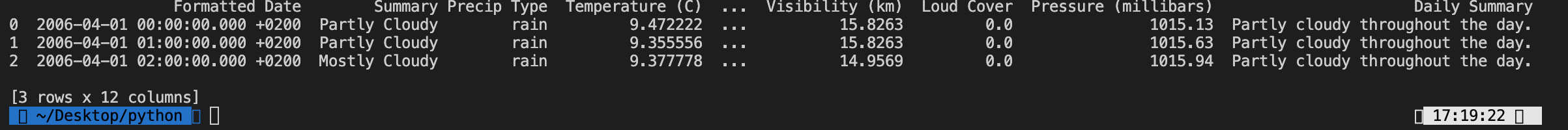查看数据信息：

weather_data.info()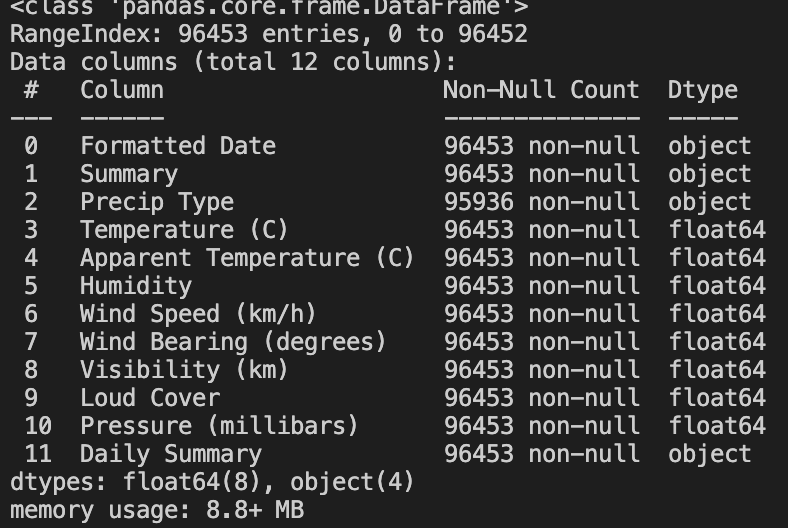查看数据集中的分类变量：

print(weather_data.describe(include='all'))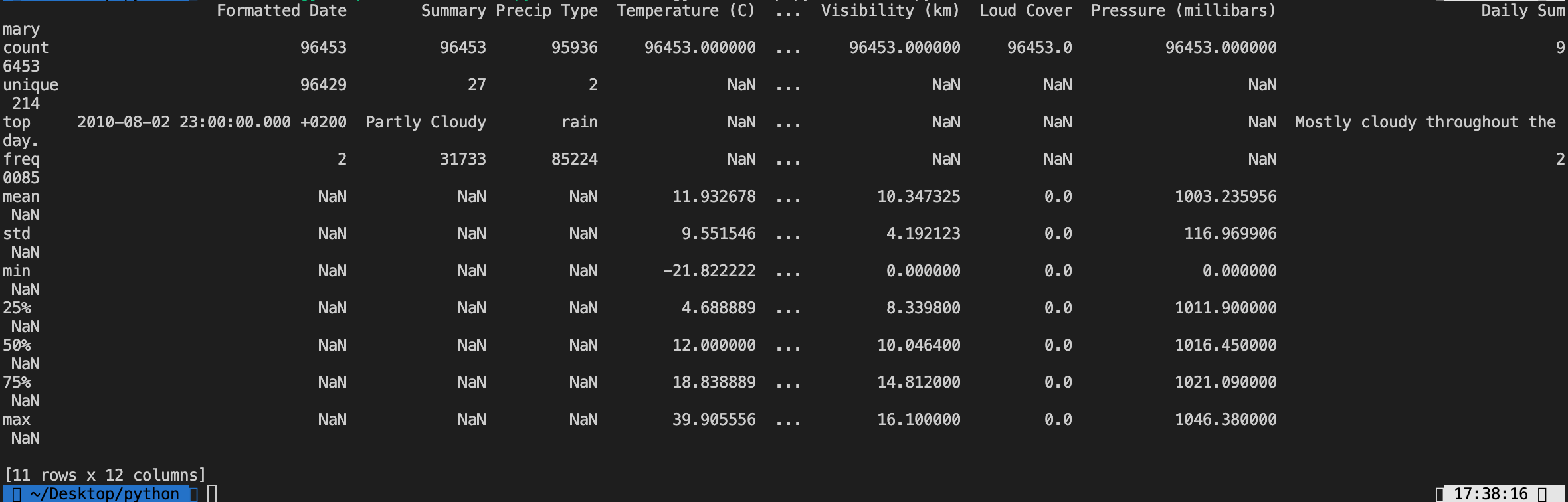print(weather_data.describe(include=['O']))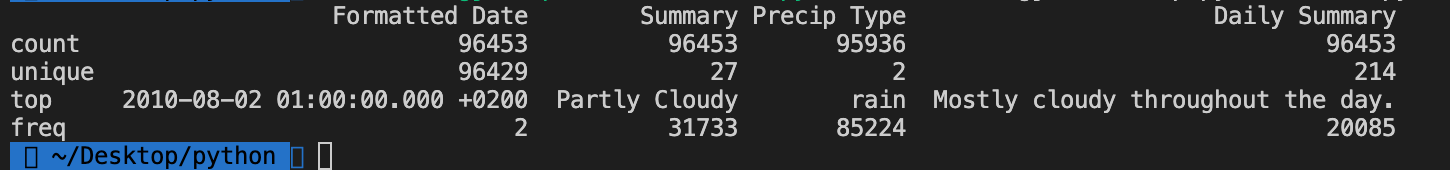要预测温度，所以需要找出数据集中不同变量之间的相关性，代码如下：

print(weather_data.corr())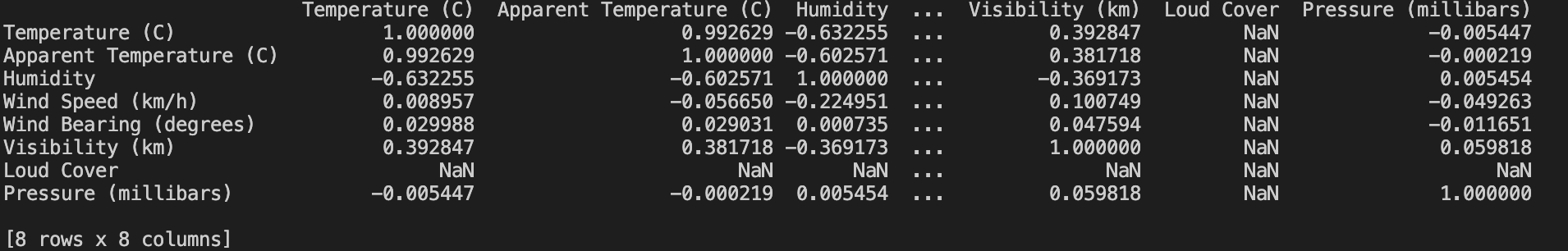相关性在-1到1之间变化，1表示强相关，相关系数-1表示完全负相关，相关系数为0表示变量之间不存在关系。从上图可知，我们看到温度与表观温度、湿度之间有很强的关系，也许我们还可以包括能见度。接着，我们将所有相关属性放入一个新的数据集中并再次检查相关性。

data_set=weather_data.iloc[:,[0,3,4,5,8]]
print(data_set.corr())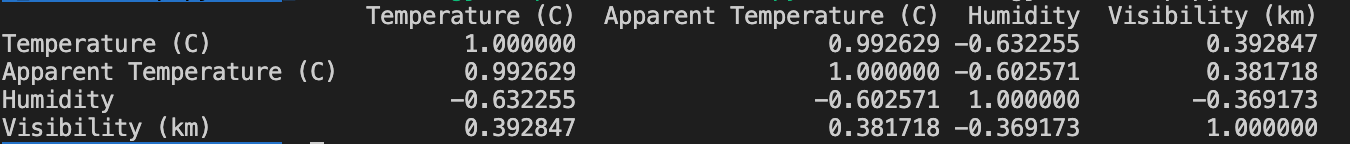现在，让我们可视化温度和其他因变量之间的数据，绘制温度和湿度之间的散点图。实现代码如下：

# 指定绘图对象的宽度和高度
plt.figure(figsize=(14,4))
plt.xlabel('Temperature (C)')
plt.ylabel('Humidity')

# 绘制温度和湿度散点图
sns.regplot(x=data_set["Temperature (C)"], y=data_set["Humidity"],data=data_set)
plt.show()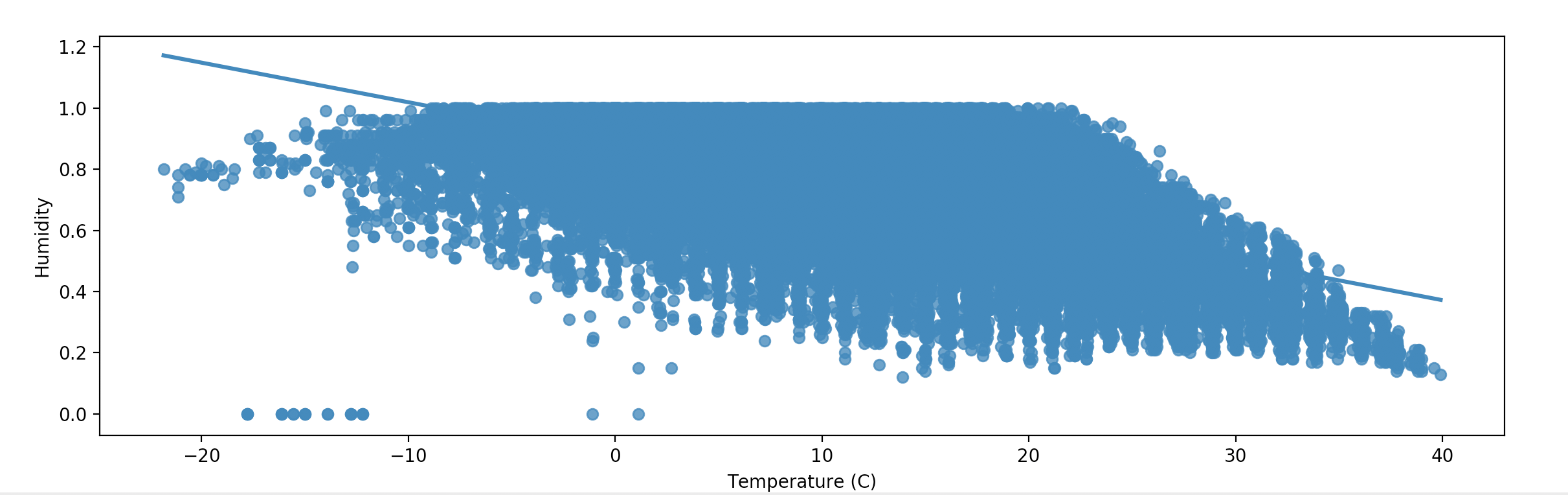图中，湿度和温度之间存在负相关，我们也看到了一些异常值。我们可以尝试找到这些异常值，并将这些异常值进行删除。在统计中了解到，异常值会极大的影响线性回归。下面，我们通过编写一个函数来帮助我们识别数据集中湿度变量的异常值。它发现异常值的方式是基于标准差大于3。实现代码如下所示：

outliers=[]
def detect_outlier(data_1):

threshold=3
mean_1 = np.mean(data_1)
std_1 =np.std(data_1)

for y in data_1:
z_score= (y - mean_1)/std_1
if np.abs(z_score) > threshold:
outliers.append(y)
return outliers

outlier_data = detect_outlier(data_set["Humidity"])
print (outlier_data)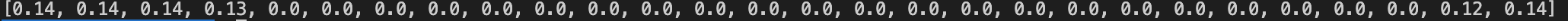接着，我们从数据集中删除这些行以获得用于回归的干净数据集。因此，我们在data_set中搜索所有值大于0.15的湿度值并创建一个新的数据集data_set_clean。实现代码如下：

data_set_clean = data_set[data_set["Humidity"]>0.15]

# 绘制干净数据的温度和湿度的散点图
sns.regplot(x=data_set_clean["Temperature (C)"], y=data_set_clean["Humidity"])
plt.show()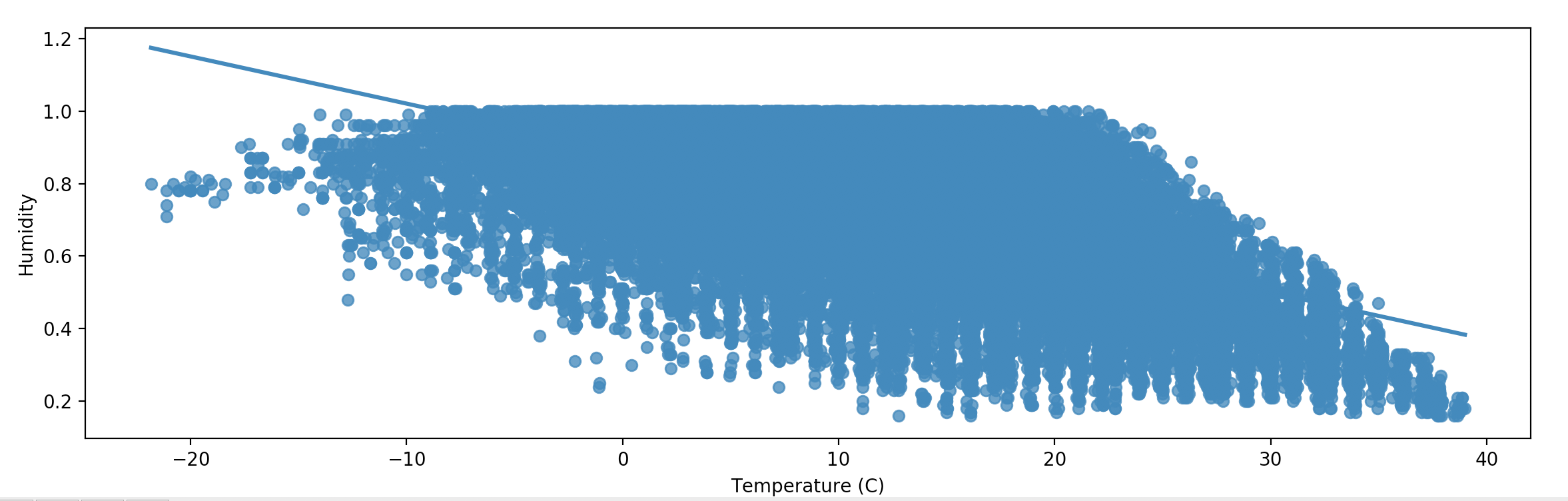现在，让我们在温度和表观温度之间再画一个散点图，实现代码如下：

# 温度与表观温度的散点图
sns.regplot(x=data_set["Temperature (C)"], y=data_set["Apparent Temperature (C)"])
plt.show()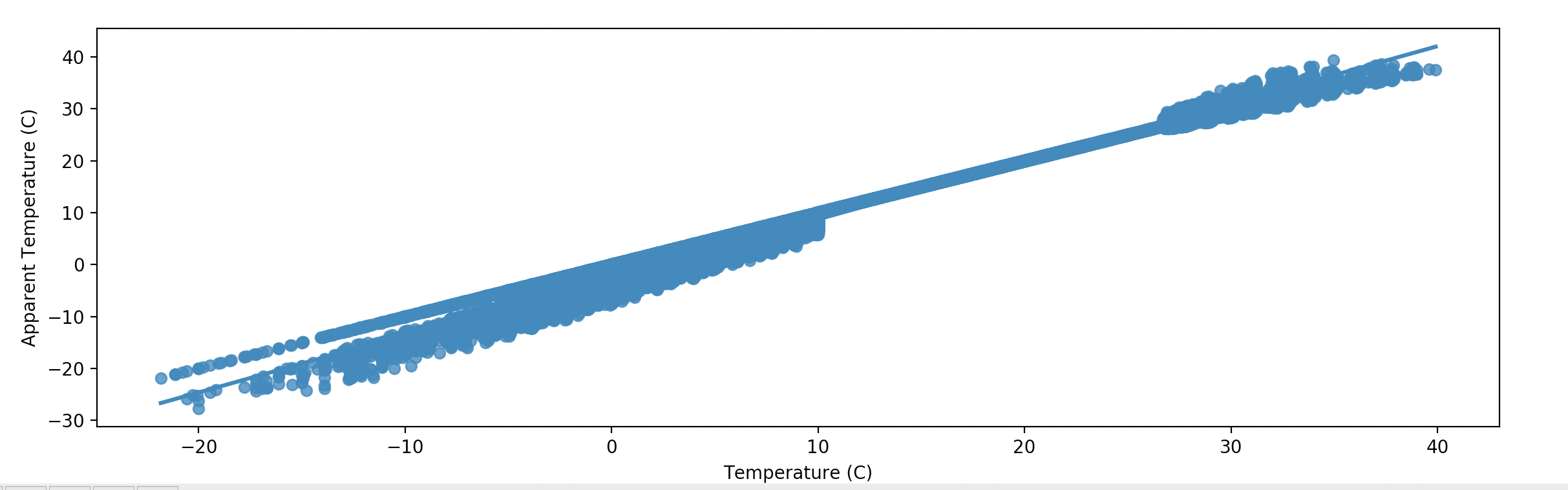从上图看起来温度和表观温度之间存在很强的正相关性，这也很明显。下面，我们再绘制温度和能见度之间的散点图，实现代码如下：

# 温度与能见度散点图
sns.regplot(x=data_set["Temperature (C)"], y=data_set["Visibility (km)"])
plt.show()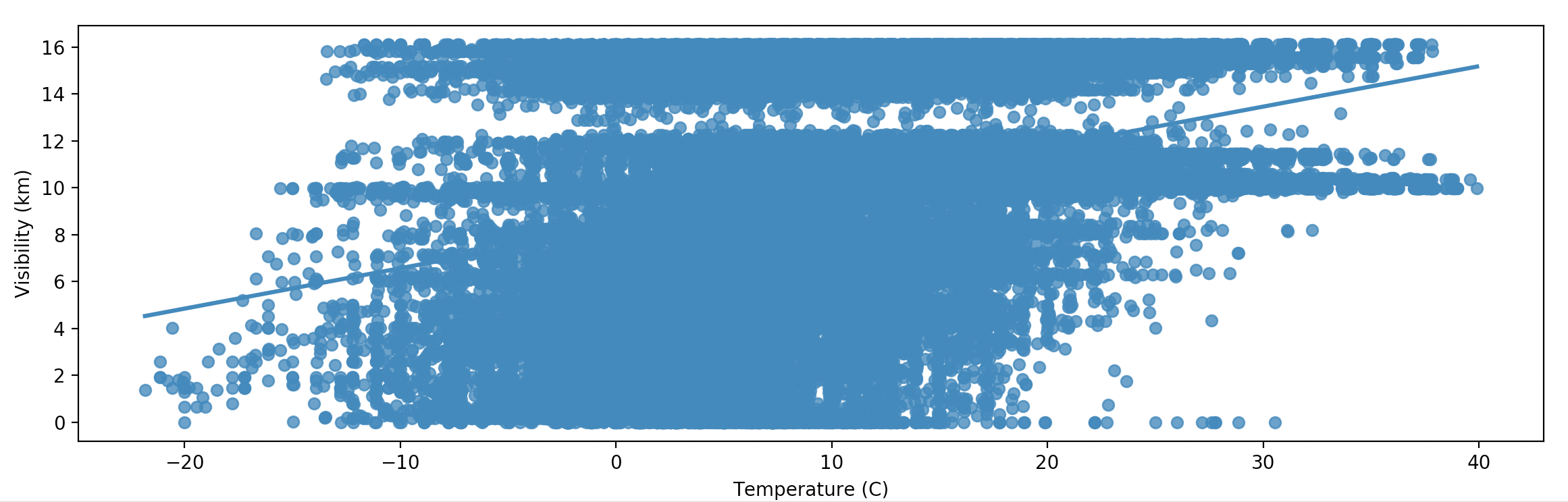从图中，我们没有看到温度和能见度之间有很强的关系，所以我们可以降低能见度。这里X是我们与湿度和表观温度的自变量，Y是我们试图首先学习然后计划预测的温度因变量。代码如下：

# 过滤数据集
data_set_clean = data_set[data_set["Humidity"]>0.15]
y= data_set_clean.iloc[:,]
X= data_set_clean.iloc[:,[2,3]]
print(data_set_clean[data_set_clean["Humidity"]<=0.15])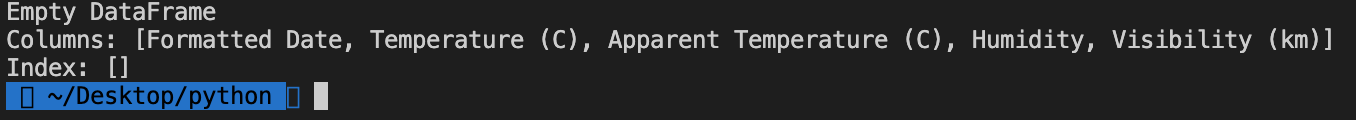打印一行X以查看我们的自变量，代码如下：

print(X.head(1))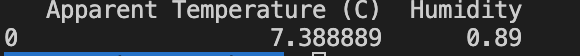通过，80:20的比例将数据集拆分为训练集和测试集，代码如下：

# 80:20 进行拆分
from sklearn.model_selection import train_test_split
X_train, X_test, y_train, y_test = train_test_split(X, y, test_size = 0.2, random_state = 0)

# 训练集
from sklearn.linear_model import LinearRegression
regressor =LinearRegression()
regressor.fit(X_train, y_train)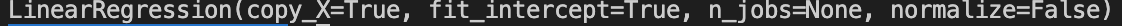接着，我们打印回归量的不同值并了解它们的含义。代码如下：

print(regressor.coef_)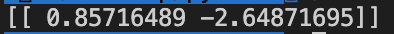记住线性回归方程式，公式如下：•  0.857是b1，其中x1是表观温度
• -2.648是b2，其中x2是湿度

print(regressor.intercept_)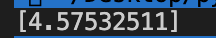现在，让我们把这些数字带入公式内进行计算，公式如下：

温度 = 4.58 + (表观温度 * 0.857) + (-2.648 * 湿度)

# 预测测试集结果
y_pred = regressor.predict(X_test)


那我们如何知道衡量模型的适用性呢？一个好的拟合模型是这样一种模型，其中实际值或观察值与基于模型的预测值之间的差异很小且无偏。所以，如果一些统计数据告诉我们实际值和预测值之间的R2可以衡量模型或回归线对实际结果的复制程度，这是基于模型解释的预测的总变化。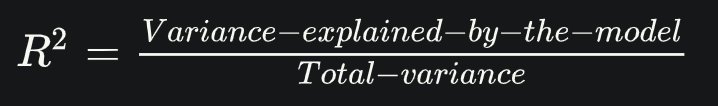R2始终介于0和1之间或者0%到100%之间。值1表示该模型解释了预测变量围绕其均值的所有变化。

• SSE = 实际值 - 预测值，表示与实际值相比，预测的值有多远；
• SST = 实际值 - 平均值，表示实际值与平均值相比有多远；
• SSR = 预测值 - 平均值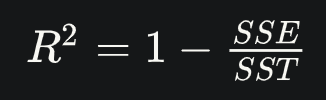如果预测误差较低，则SSE将较低且R2将接近1。这里需要注意的是，当我们添加更多的自变量时，R2会获得更高的值，R2值随着更多的自变量的加入而不断增加，即使它们可能不会真正对预测产生重大影响。为了解决这个问题，我们对R2进行了调整，调整后R2会在每次添加一个无关紧要的自变量时修正该模型。

print(regressor.score(X,y))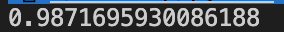RMSE显示预测值和实际值之间的变化，由于预测值和实际值之间的差异可以是正值也可以是负值，为了抵消这种差异，我们取预测值和实际值之间的差异的平方。

1. 找出每个观测值的预测值和实际值之间的差异，然后将值的平方并累加：sum(预测值-实际值)2
2. 总和除以观察次数：sum(预测值-实际值)2/观察次数
3. 取第2步中的值的平方根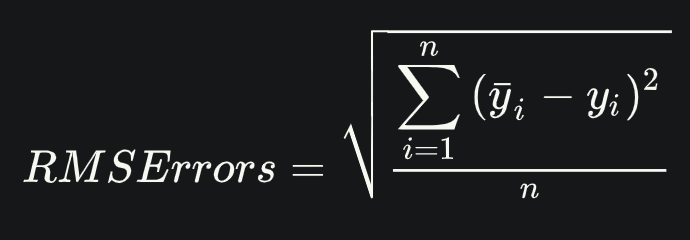实现代码如下：

from sklearn import metrics
import math
print(math.sqrt(metrics.mean_squared_error(y_test, y_pred)))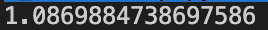接着，我们可以使用另一个库statsmodel，代码如下：

import statsmodels.api as sm
ones_1 = * X.count()
X["b0"]=ones_1

model = sm.OLS(y_pred,X_test).fit()
print(model.summary())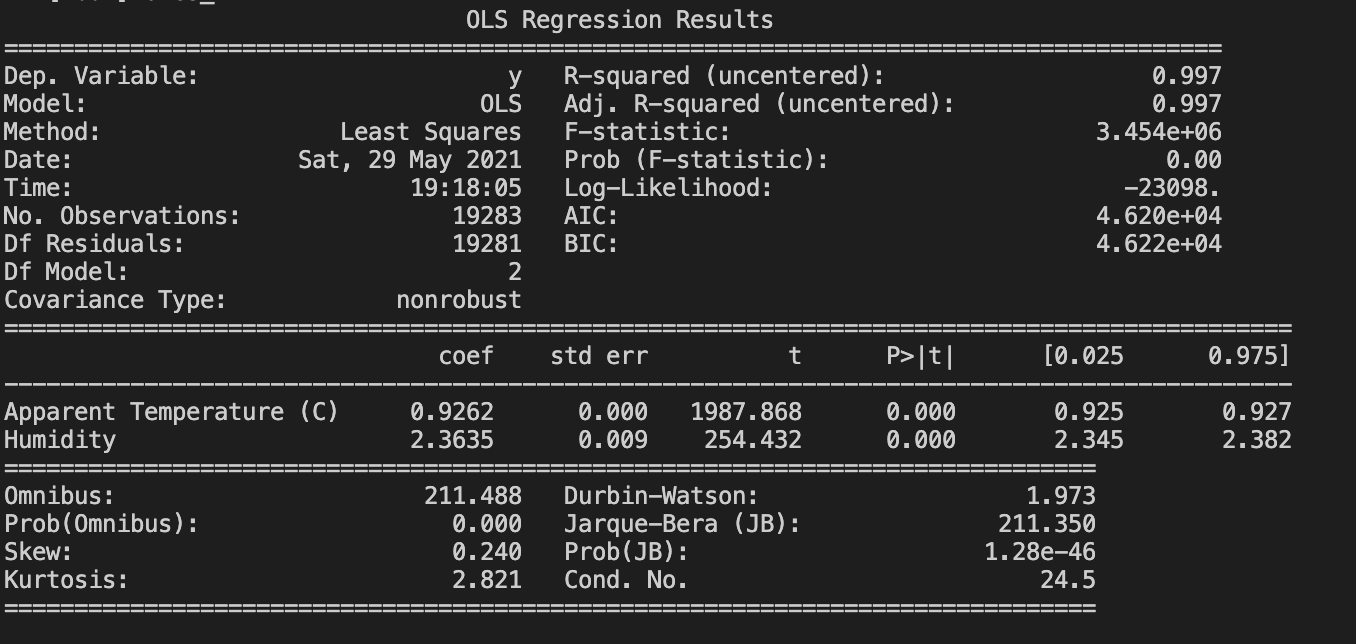从上图运行结果可以看出，我们从之前的0.987值得到了一个更加好的0.997平方根值。

# 3.总结import pandas as pd
import numpy as np
import seaborn as sns
import matplotlib.pyplot as plt

# weather_data.info()

# print(weather_data.describe(include='all'))
# print(weather_data.describe(include=['O']))
# print(weather_data.corr())

data_set=weather_data.iloc[:,[0,3,4,5,8]]
# print(data_set.corr())

# 指定绘图对象的宽度和高度
plt.figure(figsize=(14,4))
plt.xlabel('Temperature (C)')
plt.ylabel('Humidity')

# 绘制温度和湿度散点图
# sns.regplot(x=data_set["Temperature (C)"], y=data_set["Humidity"],data=data_set)
# plt.show()

outliers=[]
def detect_outlier(data_1):

threshold=3
mean_1 = np.mean(data_1)
std_1 =np.std(data_1)

for y in data_1:
z_score= (y - mean_1)/std_1
if np.abs(z_score) > threshold:
outliers.append(y)
return outliers

outlier_data = detect_outlier(data_set["Humidity"])
# print (outlier_data)

data_set_clean = data_set[data_set["Humidity"]>0.15]

# 绘制干净数据的温度和湿度的散点图
# sns.regplot(x=data_set_clean["Temperature (C)"], y=data_set_clean["Humidity"])
# plt.show()

# 温度与表观温度的散点图
# sns.regplot(x=data_set["Temperature (C)"], y=data_set["Apparent Temperature (C)"])
# plt.show()

# 温度与能见度散点图
# sns.regplot(x=data_set["Temperature (C)"], y=data_set["Visibility (km)"])
# plt.show()

# 过滤数据集
data_set_clean = data_set[data_set["Humidity"]>0.15]
y= data_set_clean.iloc[:,]
X= data_set_clean.iloc[:,[2,3]]
# print(data_set_clean[data_set_clean["Humidity"]<=0.15])

# 80:20 进行拆分
from sklearn.model_selection import train_test_split
X_train, X_test, y_train, y_test = train_test_split(X, y, test_size = 0.2, random_state = 0)

# 训练集
from sklearn.linear_model import LinearRegression
regressor =LinearRegression()
regressor.fit(X_train, y_train)
# print(regressor.coef_)
# print(regressor.intercept_)

# 预测测试集结果
y_pred = regressor.predict(X_test)
# print(regressor.score(X,y))
from sklearn import metrics
import math
# print(math.sqrt(metrics.mean_squared_error(y_test, y_pred)))

import statsmodels.api as sm
ones_1 = * X.count()
X["b0"]=ones_1

model = sm.OLS(y_pred,X_test).fit()
print(model.summary())
View Code

# 4.结束语

QQ群（Kafka并不难学）： 825943084

### 转载请注明出处，谢谢合作！

posted @ 2021-05-29 19:23  哥不是小萝莉  阅读(283)  评论(1编辑  收藏  举报